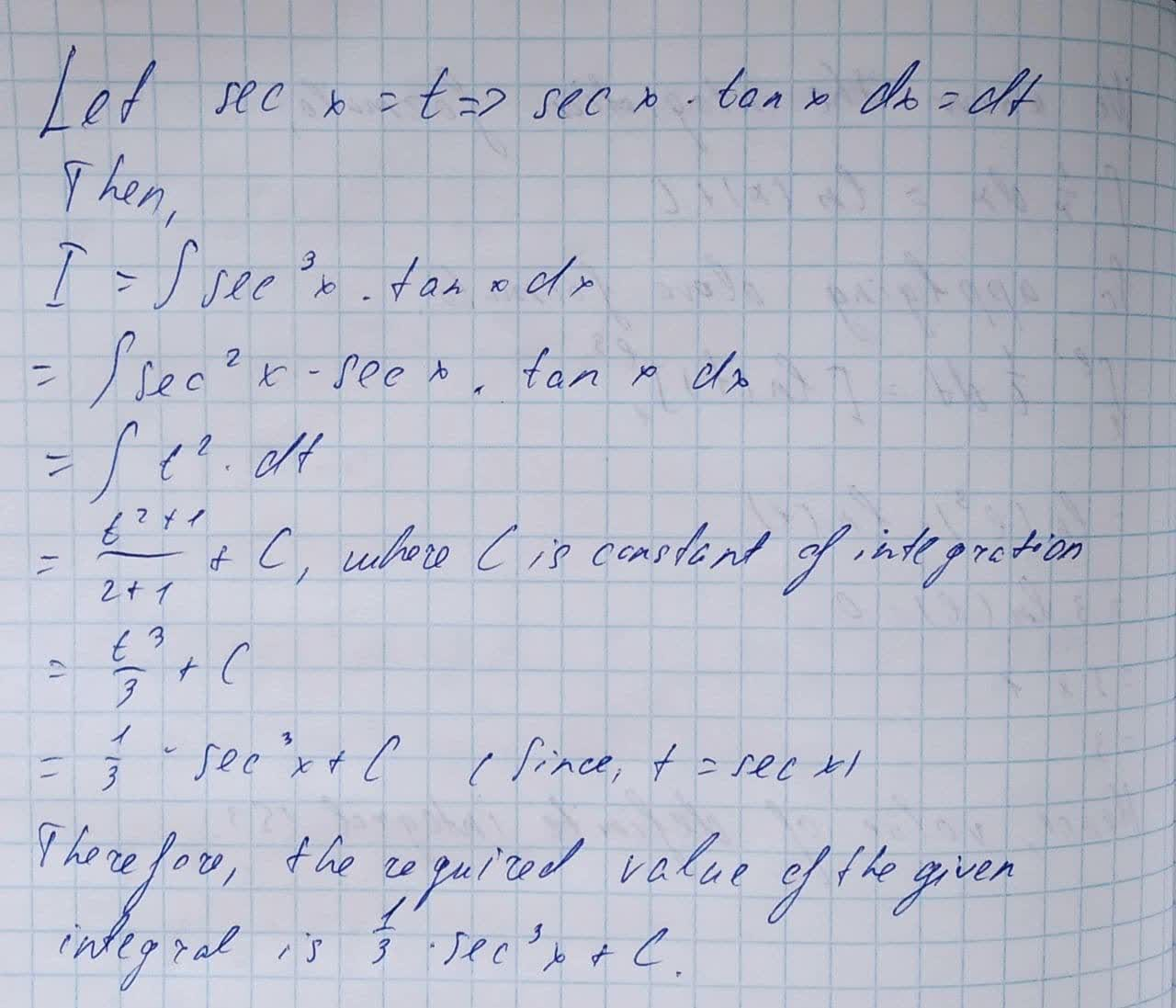Question# Evaluate the indefinite integral. \int sec^{3} x \tan x dx

Applications of integrals
ANSWEREDEvaluate the indefinite integral.
$$\displaystyle\int{{\sec}^{{{3}}}{x}}{\tan{{x}}}{\left.{d}{x}\right.}$$